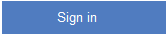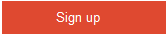# Can you explain equivalence partitioning?

–1 vote
8 viewsposted Apr 15
Share this question## 1 Answer

0 votes

In equivalence partitioning we identify inputs which are treated by the system in the same way and produce the same results. You can see from the following figure applications TC1 and TC2 give the same results (i.e., TC3 and TC4 both give the same result, Result2). In short, we have two redundant test cases. By applying equivalence partitioning we minimize the redundant test cases.
Software Testing Image
So apply the test below to see if it forms an equivalence class or not:

``````All the test cases should test the same thing.
They should produce the same results.
If one test case catches a bug, then the other should also catch it.
If one of them does not catch the defect, then the other should not catch it.
``````answer Apr 16
Similar Questions